# # Reduction

## # Overview

The ReductionProcessor takes an input scene and reduces the number of triangles and vertices in the scene in a heuristic manner by gradually removing vertices and triangles from the scene.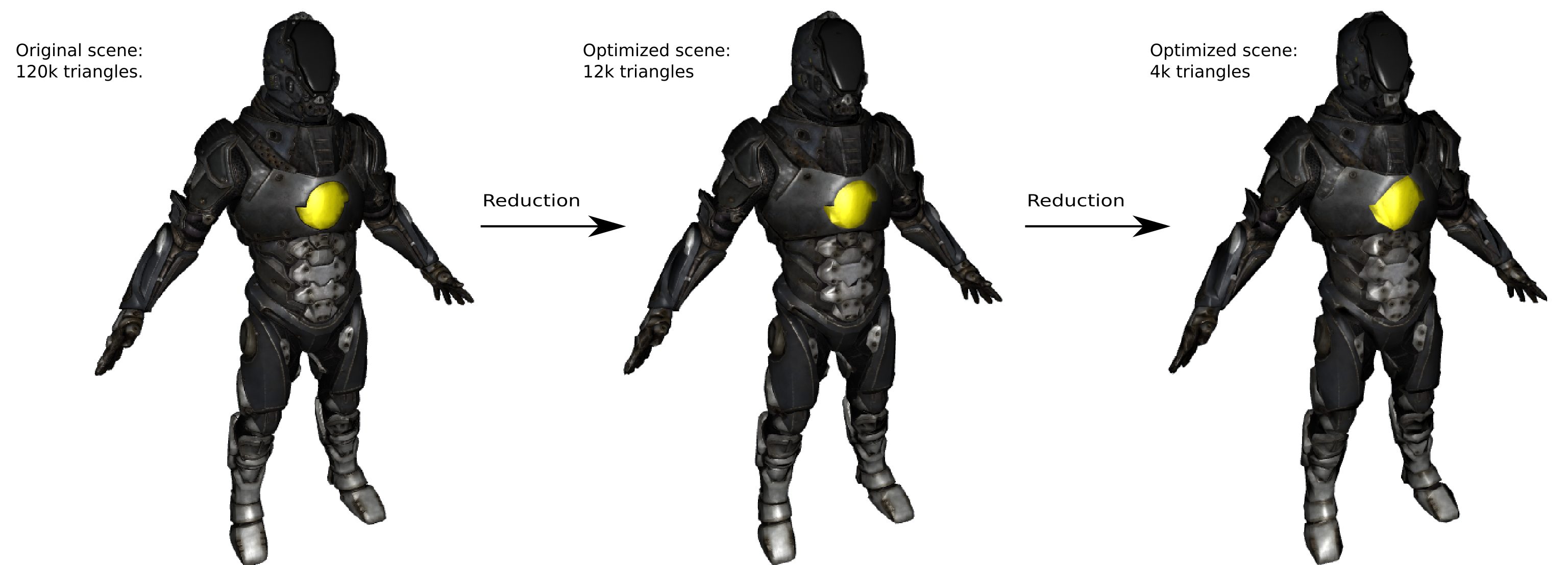## # Feature importance

When reducing a geometry, there are plentty of different data that needs to be taken into consideration. The most obvious one being purely geometrical features of the mesh, but also things like UV coordinates, tangents, normals, vertex colors, and skinning are also important to preserve. In Simplygon, the user can weight how important each different feature is individually so that they get a higher priority relative to the others.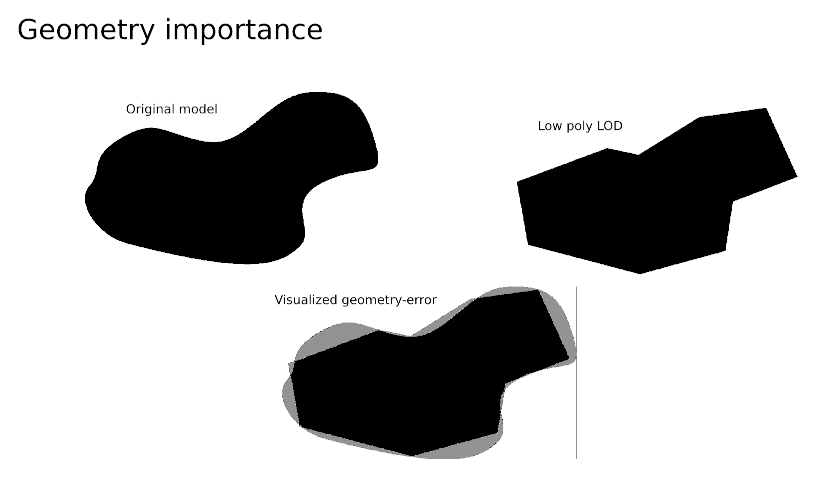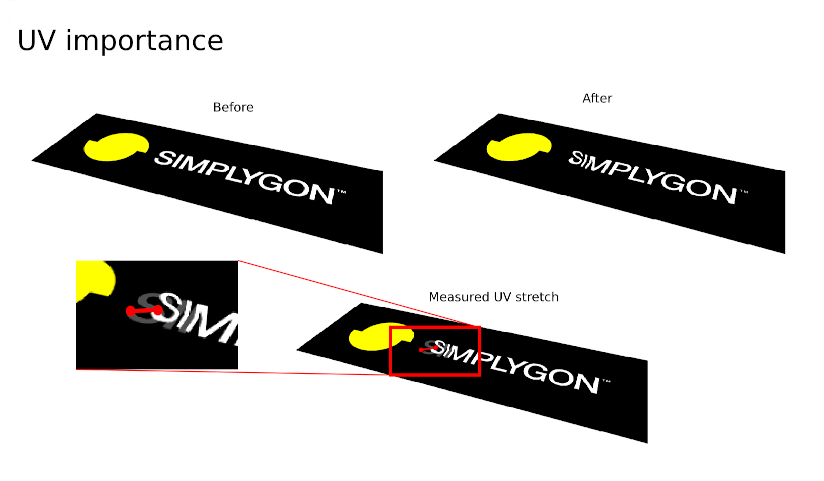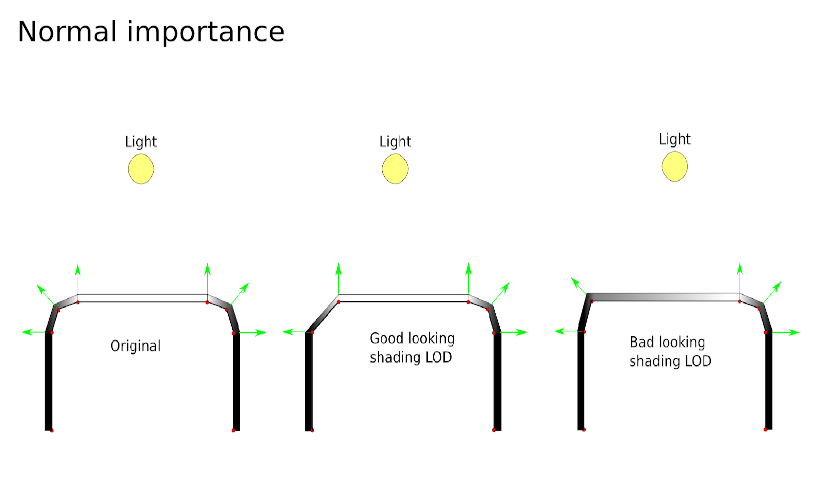## # Targets and stop conditions

The reduction runs until one or multiple criterias have been met. The target can be a specific triangle count or ratio. But it can also be be set to a maximum deviation threshold or set to be optimized for a specific on screen size - meaning the remaining triangle count won't be known prior to reduction, but the quality will.

## # Geomorph

When creating a LOD with the Reduction Processor, Simplygon has the possibility to create a Geomorph model for the original model. This Geomorph model contains the same number of triangles as the original model, and contains all corner / vertex data needed to morph the original into looking like the LOD. Usually this data are the common fields such as vertex colors, UVs, normals, tangents, bone-weights etc, but it can be any data fields that has been loaded into the Geometry. Using Geomorph right before swapping between the models gives a smooth and pop-free LOD transition.## # Symmetry

Simplygon detects if a mesh is symmetrical and mirrors the reduction on both sides of the symmetry plane. Symmetric re-triangulation creates a symmetry in the triangulation, where possible, of a mesh before processing. Typically, this is useful when working with triangulated quad meshed, where symmetric features are present, but where triangulation is asymmetric with respect to some given plane.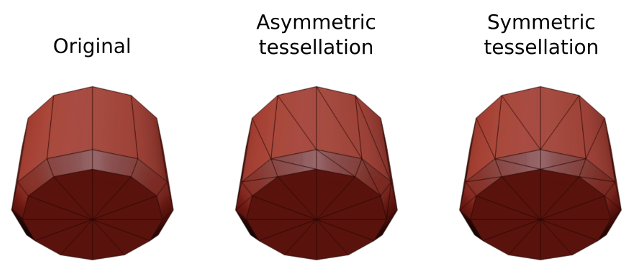## # Supported components

The Reduction processor works with: